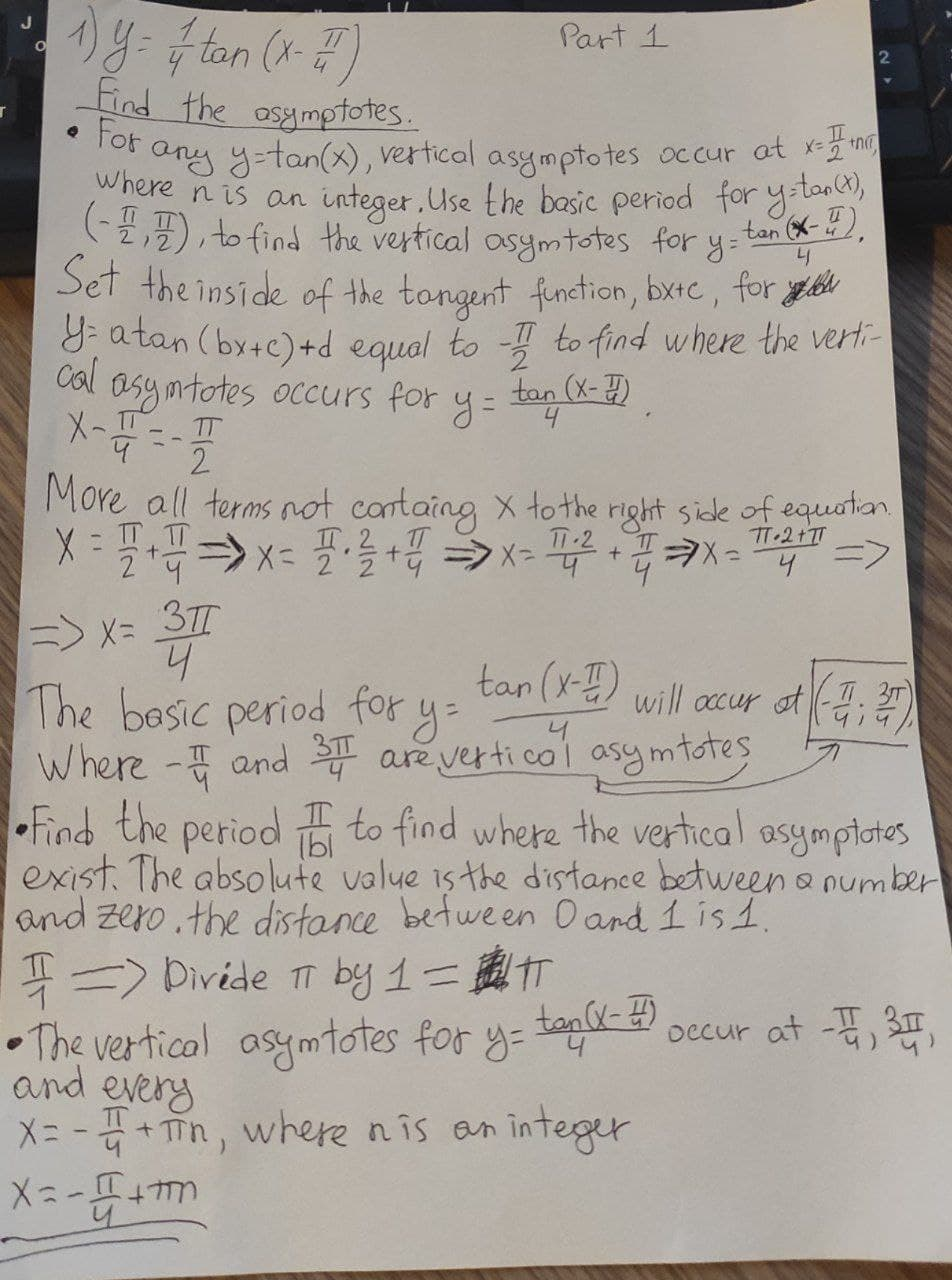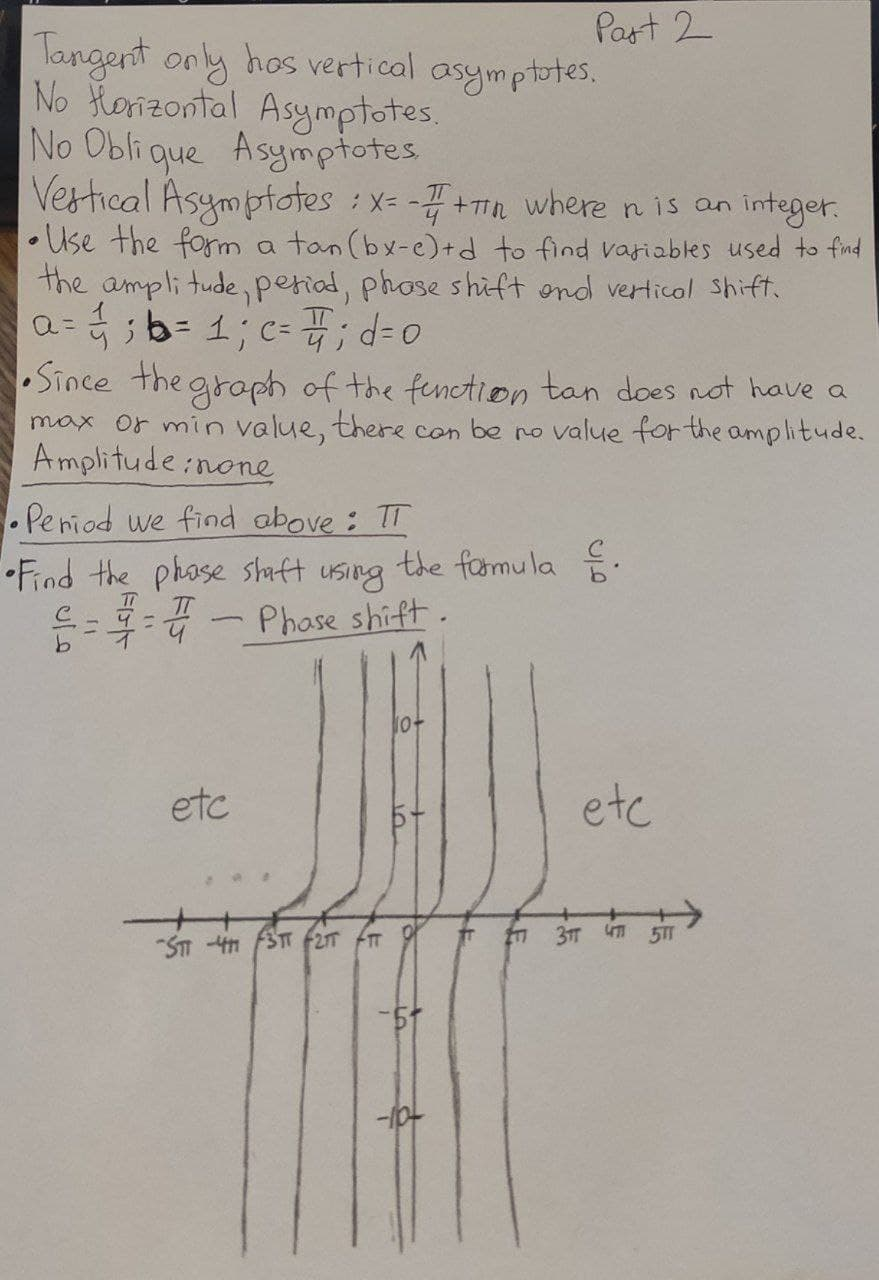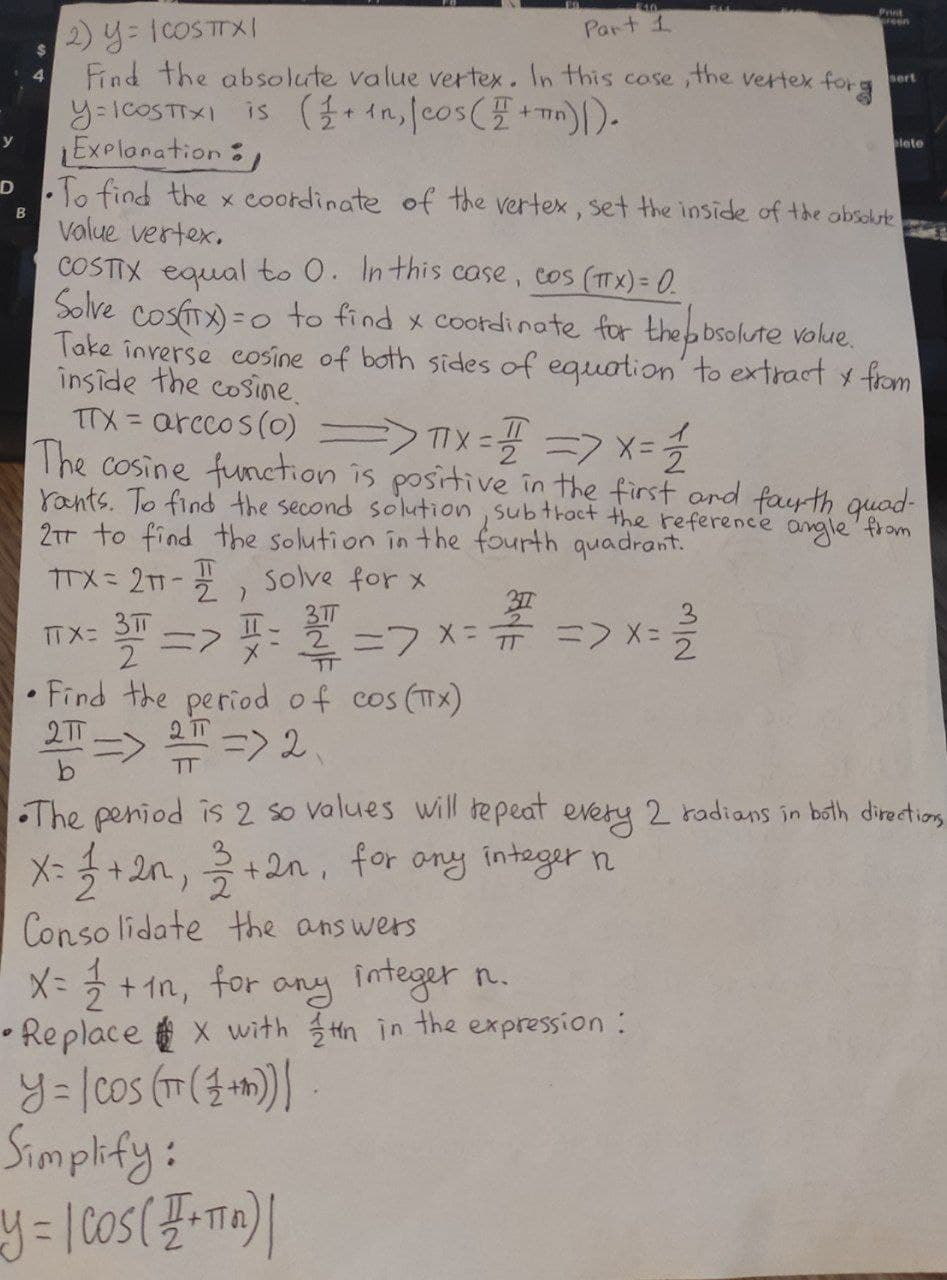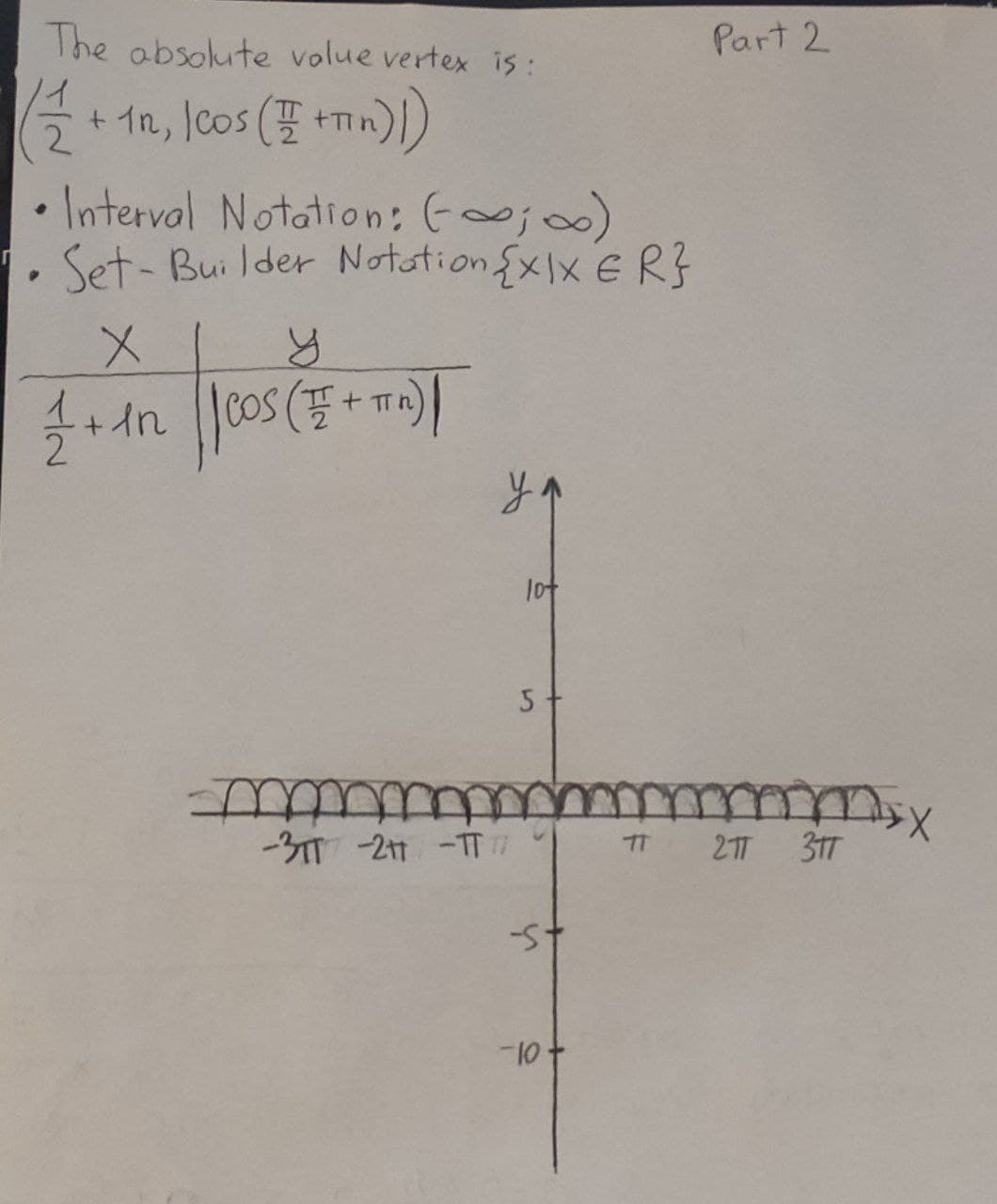# Graph the following functions by hand, not by plotting the points, but by starting with the graph of one of the standard functions and then applying the appropriate transformations

Graph the following functions by hand, not by plotting the points, but by starting with the graph of one of the standard functions and then applying the appropriate transformations y=1/4tan(x-Pi/4) y=|cosPix|
You can still ask an expert for help

• Questions are typically answered in as fast as 30 minutes

Solve your problem for the price of one coffee

• Math expert for every subject
• Pay only if we can solve itnick1337

Here is graph and explanation: